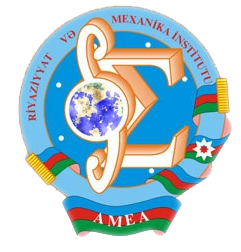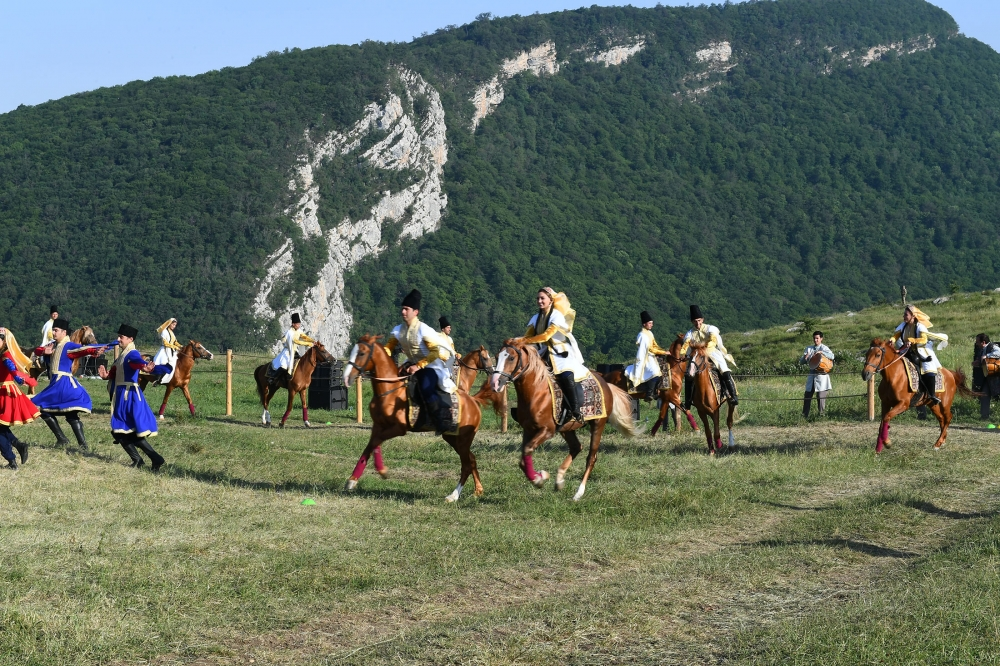Riyaziyyat və Mexanika İnstitutu

## Qarabağ xəbərləri### Şuşada Heydər Əliyev Fondunun təşkilatçılığı ilə “Musiqi irsi və Qarabağ atları Cıdır düzündə” adlı kompozisiya təqdim olunub## Proceedings 2005/22/XXX

 Year: 2005 Volume: XXII Number: XXX MATHEMATICS Akhmedova E.N. Abstract [PDF] The definition of one class of Sturm-Liouville operators with discontinuous coefficients by Weyl function [PDF] 3 Aliev A.B., Kazimov A.A. Abstract [PDF] On behaviour of solutions of Cauchy problem for one class Sobolev type equations [PDF] 9 Babayev Sh.A. Abstract [PDF] On finding of generating function of steps number of some class semi Markovprocess with upper delaying screen [PDF] 17 Bilalov B.T., Muradov T.R. Abstract [PDF] Defective bases of Banach spaces [PDF] 23 Caksu A.G., Aslim G. Abstract [PDF] b-I-open sets and decomposition of continuity via idealization [PDF] 27 Gasratova M.G. Abstract [PDF] A class of probability distributions in the models of moving particles [PDF] 33 Humbataliyev R.Z. Abstract [PDF] On solvability of one class of operator-differential equations of the fourth order in the weight space [PDF] 39 Ismayilov F.R. Abstract [PDF] To the scattering theory for one class Sturm-Liouville operator with discontinuous coefficients [PDF] 47 Kerimov N.B., Poladov R.G. Abstract [PDF] On Basicity in Lp (0, 1) (1 < p < 1) of the system of eigenfunctions of one boundary value problem [PDF] 53 Latifova A.R. Abstract [PDF] The inverse problem of one class of Dirac operators with discontinuous coefficients by the Weyl function [PDF] 65 Mamedova A.J. Abstract [PDF] Mixed problem for one correct by Petrovskii equation in bounded domain [PDF] 71 Mammadova Sh.N. Abstract [PDF] Stabilization of solutions of linear parabolic equations [PDF] 81 Mirzoyev S.S., Aliyeva A.T. Abstract [PDF] On solvability of one class of boundary value problem for a fourth order operator-differential equation [PDF] 85 Musayev K.M., Gasanova T.Kh. Abstract [PDF] Riemann boundary value problem in a class of generalized analytic functions [PDF] 93 Guliyev N.J. Abstract [PDF] The regularized trace formula for the Sturm-Liouville equation with spectral parameter in the boundary conditions [PDF] 99 Tahsin O. Abstract [PDF] Filtration of some well-known modal logicsm [PDF] 103 Sadykova F.S. Abstract [PDF] Approximation by the mixed bilinear forms [PDF] 117 Safarov R.A. Abstract [PDF] On influence of Schrodinger operator potential continuity module on equiconvergence rate [PDF] 121 MECHANICS Gadjiev V.D., Gasymov H.M. Abstract [PDF] Stability of non-uiform geometrically non-linear cylindrical cover subject to anisotropic resistance [PDF] 135 Isayev F.K., Bagirov R.A. Abstract [PDF] On the stability of orthotropic elasticoplastic plates with regard to cross shears [PDF] 141 Mamedzade R.A. Abstract [PDF] Mathematical modelling of nonlocal fluid filtration in subsurface linearly-hereditary media and defining the hydrodynamic parameters of stratum around running well [PDF] 145 Mekhtiyev A.I. Abstract [PDF] Mixed boundary-value problem for multiply connected piecewise-homogeneous anisotropic half-plane [PDF] 157 Najafov M.A. Abstract [PDF] Formulation of the conic cover flatter problem.Perturbed state [PDF] 163 Talybly L.Kh., Gasanova P.V. Abstract [PDF] Stress distribution at elastico-plastic deformation in a monoaxially stretched plate with circular hole [PDF] 167 APPLIED PROBLEMS OF MATHEMATICS AND MECHANICS Agayeva Ch.A. Abstract [PDF] On one stochastic optimal control problem with control delay [PDF] 171 Aliyev A.Y. Abstract [PDF] Numerical solution of one nonhomogeneous nonlocal mixed problem [PDF] 179 Allahverdiyev A.A. Abstract [PDF] The optimization method of transport problem distribution [PDF] 187 Imanova M.N. Abstract [PDF] Numerical solution of Volterra type integro-differential equation [PDF] 197
Azərbaycanda COVID-19 ilə bağlı statistika
• Virusa yoluxan

596973

• Sağalan

565521

• Yeni yoluxan

0

• Aktiv xəstə

23469

• Ölüm halı

7983

• Test edilib

5,608,158